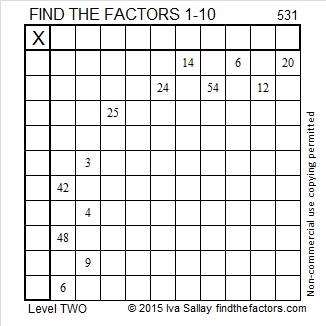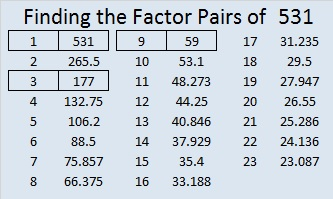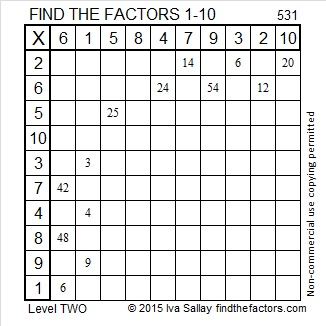# 531 and Level 2

5 + 3 + 1 = 9 so 531 is divisible by 3 and by 9. Because it can be evenly divided by 9, it’s square root can be reduced.

531 ÷ 9 = 59, a prime number which obviously has no square factors. Thus √531 = (√9)(√59) = 3√59 in its most reduced form.Print the puzzles or type the solution on this excel file: 10 Factors 2015-06-22

—————————————————————————————————

• 531 is a composite number.
• Prime factorization: 531 = 3 x 3 x 59, which can be written 531 = (3^2) x 59
• The exponents in the prime factorization are 2 and 1. Adding one to each and multiplying we get (2 + 1)(1 + 1) = 3 x 2  = 6. Therefore 531 has exactly 6 factors.
• Factors of 531: 1, 3, 9, 59, 177, 531
• Factor pairs: 531 = 1 x 531, 3 x 177, or 9 x 59
• Taking the factor pair with the largest square number factor, we get √531 = (√9)(√59) = 3√59 ≈ 23.04343724—————————————————————————————————This site uses Akismet to reduce spam. Learn how your comment data is processed.